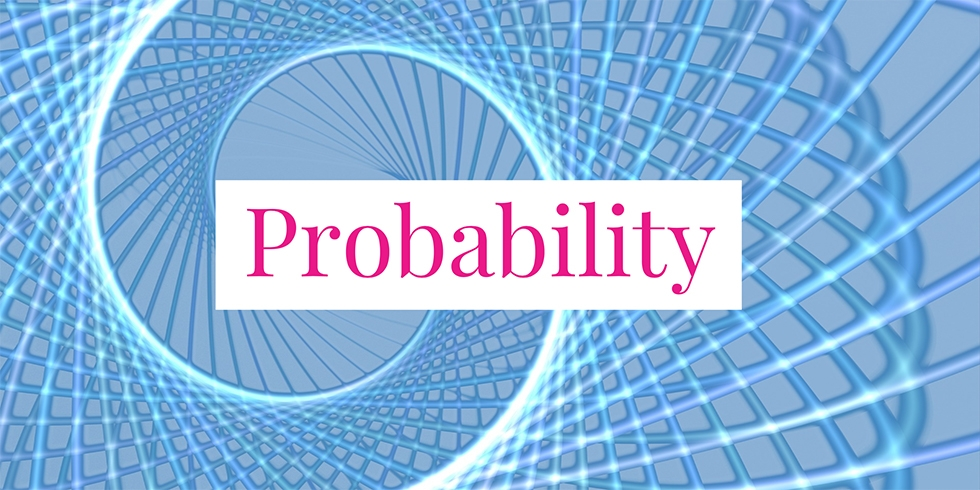# Probabilities of An Infinite Sequence of Die Rolling## Problem 745

Consider an infinite series of events of rolling a fair six-sided die. Assume that each event is independent of each other. For each of the below, determine its probability.

(1) At least one die lands on the face 5 in the first $n$ rolls.
(2) Exactly $k$ dice land on the face 5 in the first $n \geq k$ rolls.
(3) Every die roll results in the face 5.Add to solve later

## Solution.

### Solution of (1)

Let $E_i$ be the $i$th die roll event that lands on the face 5. Then we have the probability $P(E_i) = 1/6 =: p$. Then the desired probability is obtained as follows.
\begin{align*}
&P(\text{at least one 5 in the first $n$ trials}) \\
&= 1 – P(\text{no 5 in the first $n$ trials})\\
&= 1 – P(E_1^c E_2^c \cdots E_n^c)\\
&= 1 – P(E_1^c) P(E_2^c) \cdots P(E_n^c) && \text{ by independence}\\
&= 1 – \left(1-P(E_1)\right) \left(1-P(E_2)\right) \cdots \left(1-P(E_n)\right)\\
&= 1 – (1-p)^n\\
&= 1 – (5/6)^n
\end{align*}

### Solution of (2)

Suppose that we have exactly $k$ dice land on the face 5 and the rest of $n-k$ trials land other than 5. The probability of such an event is given by $p^k(1-p)^{n-k}$ as each trial is independent of each other. Now, there are totally ${n \choose k}$ such sequences of events. Thus, the probability that exactly $k$ dice land on the face 5 in the fist $n$ rolls is
${n \choose k}p^k(1-p)^{n-k} = {n \choose k}\left(\frac{1}{6}\right)^k \left(\frac{5}{6}\right)^{n-k} = {n \choose k} \frac{5^{n-k}}{6^n}.$

### Solution of (3)

Let $F_i$ be the event that all die rolls land the face 5 for the first $i$th trials. Thus, $F_i = E_1 E_2 \cdots E_i$. Note that the sequence $\{F_i\}$ is increasing, that is, we have
$F_1 \subset F_2 \subset \cdots \subset F_n \subset \cdots.$

Now, the desired probability can be expressed as $P(\lim_{n\to \infty} F_n)$. By the result of Problem “Interchangeability of Limits and Probability of Increasing or Decreasing Sequence of Events“, we obtain
\begin{align*}
&P\left(\lim_{n\to \infty} F_n \right)\\
&= \lim_{n \to \infty} P(F_n)\\
&= \lim_{n \to \infty} P(E_1 E_2 \dots E_n)\\
&= \lim_{n \to \infty} P(E_1) P(E_2) \cdots P(E_n) && \text{ by independence}\\
&= \lim_{n \to \infty} p^n \\
&= \lim_{n \to \infty} (1/6)^n\\
&= 0.
\end{align*}
Therefore the probability that all die rolls result in the face 5 is zero.Add to solve later

### More from my site

#### You may also like...

This site uses Akismet to reduce spam. Learn how your comment data is processed.

###### More in Probability##### Interchangeability of Limits and Probability of Increasing or Decreasing Sequence of Events

A sequence of events $\{E_n\}_{n \geq 1}$ is said to be increasing if it satisfies the ascending condition \[E_1 \subset...

Close